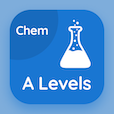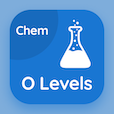Online A Level Courses

A Level Chemistry Practice Tests

A Level Chemistry Online Tests

The Book What is Organic Chemistry Multiple Choice Questions (MCQ Quiz) with answers, What is Organic Chemistry MCQ Quiz PDF download to study online a level chemistry courses. Practice Introduction to Organic Chemistry Multiple Choice Questions and Answers (MCQs), What is Organic Chemistry quiz answers PDF for SAT test prep classes. The eBook What is Organic Chemistry MCQ App Download: stereoisomerism, what is organic chemistry test prep for completely online college.

The MCQ: The substances which are the basis of human life on earth are PDF, "What is Organic Chemistry" App Download (Free) with atom, molecules, matter, and organic compounds choices for SAT test prep classes. Study what is organic chemistry quiz questions, download Google eBook (Free Sample) for completely online college.

## Chemistry MCQs: What is Organic Chemistry Quiz Questions

MCQ: The substances which are the basis of human life on earth are

A) atom
B) molecules
C) matter
D) organic compounds

MCQ: For complex molecules, a chemist usually represents a molecule by

A) molecular formula
B) empirical formula
C) skeletal formula
D) simple formula

MCQ: The element that is the backbone of organic molecules is

A) carbon
B) hydrogen
C) oxygen
D) nitrogen

MCQ: The most simplified version of the displayed formula is called

A) molecular formula
B) empirical formula
C) structural formula
D) skeletal formula

MCQ: The actual numbers of every type of molecule which is present in a compound are represented by

A) molecular formula
B) empirical formula
C) structural formula
D) skeletal formula

### Practice Tests: A Level Chemistry Exam Prep

Download A level Chemistry Quiz App, O Level Chemistry MCQ App, and SAT Chemistry MCQs App to install for Android & iOS devices. These Apps include complete analytics of real time attempts with interactive assessments. Download Play Store & App Store Apps & Enjoy 100% functionality with subscriptions!